# Test: General Intelligence & Reasoning- 3

## 50 Questions MCQ Test Mock Test Series for SSC JE Electrical Engineering | Test: General Intelligence & Reasoning- 3

Description
Attempt Test: General Intelligence & Reasoning- 3 | 50 questions in 50 minutes | Mock test for SSC preparation | Free important questions MCQ to study Mock Test Series for SSC JE Electrical Engineering for SSC Exam | Download free PDF with solutions
QUESTION: 1

### Select the related letters from the given alternatives. MANISHA : MNSA :: RAVINA : ?

Solution:

In this question, the odd alphabets are chosen, i.e. MANISHA ⇒ 1st 3rd 5th 7th = MNSA, therefore RAVINA will give us RVN.

QUESTION: 2

### Select the related letters from the given alternatives. QSUW : TVXZ :: ACEG : ?

Solution:

The analogy is addition of 3 characters to each of the alphabets.

Q + 3 = T,

S + 3 = V,

U + 3 = X,

W + 3 = Z

Similarly,

A + 3 = D,

C + 3 = F,

E + 3 = H,

G + 3 = J

Thus, ACEG will become DFHJ.

QUESTION: 3

### Select the related number from the given alternatives. 14 : 30 : : 16 : ?

Solution:

In 14 : 30 ⇒ 14 : (14 + 1) × 2.

Similarly,

In 16 : ? ⇒ 16: (16 + 1) × 2 ⇒ 16 : 34

QUESTION: 4

Select the related number from the given alternatives.

111 : 222 :: 444 : ?

Solution:

There is difference of 1 between each digit of 111 and 222.

Similarly, there will be difference of 1 between each digit of 444 and 333.

Hence, 333 is correct option.

QUESTION: 5

Select the related word from the given alternatives.

Pesticide : Plant :: ? : ?

Solution:

Pesticide is used to protect plants from harmful insects similarly vaccinations is given to the baby to protect the baby from harmful diseases.

Hence Vaccination is related to Baby.

QUESTION: 6

In the following question, select the related number from the given alternatives.

ABE : 8 :: KLO : ?

Solution:

The pattern followed is that the number is the sum of the position of each letter in Englishalphabetical series i.e. In ABE ⇒ A → 1; B → 2; E → 5 & 1 + 2 + 5 = 8

Similarly, In KLO ⇒ K → 11; L → 12; O → 15 & 11 + 12 + 15 = 38

QUESTION: 7

Select the related number from the given alternatives.

453 : 99 :: 642 : ?

Solution:

In 453 : 99

⇒ 453 = 4 + 5 + 3 = 12;

12 + 12/2 = 12 + 6 = 18

⇒ 99 = 9 + 9 = 18

Similarly,

⇒ 642 = 6 + 4 + 2 = 12

⇒ 12 + 12/2 = 12 + 6 = 18

1) 765 ⇒ 7 + 6 + 4 = 17

2) 346 ⇒ 6 + 4 + 3 = 13

3) 867 ⇒ 8 + 6 + 7 = 21

4) 396 ⇒ 3 + 9 + 6 = 18

Hence, 396 will be correct option.

QUESTION: 8

In the following question, select the related word from the given alternatives.

White: Green: : Grapes: ?

Solution:

White and Green are types of colors, similarly, Grapes are related to apples because both come under fruits.

QUESTION: 9

In the following question, select the related word from the given alternatives.

Garden : Plants :: Book : ?

Solution:

Here, the 1st word contains the 2nd word i.e.

Garden : Plants → Garden contains Plants.

Similarly, Book contains Pages.

Thus, Pages is the correct alternative.

QUESTION: 10

Select the related word from the given alternatives:

Crime : Court :: Disease : ?

Solution:

As the Crimes are solved in Court, in similar way Diseases are solved in Hospitals

QUESTION: 11

A series is given with one term missing. Select the correct alternative from the given ones that will complete the series.

2, 3, 5, 9, 17, ?

Solution:

Here,

2 + 1 = 3

3 + 2 = 5

5 + 4 = 9

9 + 8 = 17

17 + 16 = 33

Therefore 33 will complete the series.

QUESTION: 12

Find out the odd numbers from the given alternatives.

Solution:

Here 64 is perfect square but other three are not.

Hence, “64” is odd one among given alternatives.

QUESTION: 13

In the question, select which is different from the other three alternatives.

Solution:

Here, all the members except “Student” belong to the category of faculty.

QUESTION: 14

In the question, select the option which is different from the other three alternatives.

Solution:

Grinding Maize, Barley and wheat we can get flour while we obtain oil from mustard seeds.

QUESTION: 15

In the question below find the odd word from the given alternatives.

Solution:

Trousers are worn below the waist whereas all the others are worn above the waist.

QUESTION: 16

Find the odd letters from the given alternatives.

Solution:

In all the words the second letter is 4th to the right of first letter except in ‘MO’ where ‘O’ is 2nd letter from ‘M’.

A + 4 = E;

C + 4 = G;

J + 4 = N;

M + 2 = O

QUESTION: 17

Find the odd letters from the given alternatives.

Solution:

The pattern is as follows,

F + 3 = I, I + 2 = K

P + 3 = S, S + 2 = U

T + 3 = W, W + 2 = Y

D + 3 = G, G + 3 = J

Thus, DGJ is the odd word.

QUESTION: 18

Directions: Select the one which different from the other responses.

Solution:

All other sets contains alternate letters except MNO which is continuous series.

QUESTION: 19

Choose the odd number from the given alternatives.

Solution:

1) 243 - 132 = 111  (divisible by 3)

2) 183 - 54 = 129    (divisible by 3)

3) 108 - 97 = 11       (not divisible by 3)

4) 99 - 63 = 36       (divisible by 3)

Hence, 108 - 97 is odd one out.

QUESTION: 20

Find out the odd number from the given alternatives.

Solution:

1) 369 ⇒ 3 + 6 = 9

2) 257 ⇒ 2 + 5 = 7

3) 346 ⇒ 3 + 4 = 7

4) 628 ⇒ 6 + 2 = 8

→ Except 346, last digit of number can be obtained by adding first and second digit of number.

QUESTION: 21

In the following questions, a series is given with one or more number(s) / alphabet missing. Choose the correct alternative from the given options.

1, 1, 2, 3, 5, 8, ?

Solution:

This is Fibonacci series in which each term is obtained by adding previous two terms. Therefore, the required number is 8 + 5 = 13.

QUESTION: 22

In the question given below find the wrong term?

12, 24, 36, 40, 48

Solution:

12 = 6 × 2

24 = 6 × 4

36 = 6 × 6

40 = not a multiple of 6

48 = 6 × 8

Hence 40 is the wrong term.

QUESTION: 23

Select the missing number from the given responses.

3, 3, 6, 18, 72, 360, ?

Solution:

Given series is as follows

⇒ 3 × 1 = 3

⇒ 3 × 2 = 6

⇒ 6 × 3 = 18

⇒ 18 × 4 = 72

⇒ 72 × 5 = 360

Similarly,

⇒ 360 × 6 = 2160

QUESTION: 24

Which of the following words follow the trend of the given list?

Vertex, Affix, Unix, Waxy, Extra,?

Solution:

Here We need to observe the position of 'x'. 'Vertex' has letter 'x' at the sixth position, 'Affix' has it at fifth, 'Unix' at fourth, Waxy at third and 'extra' at second.

Hence answer should be the word wherein 'x' is at the first position.

Hence Option 2 is the correct answer.

QUESTION: 25

A series is given with one term missing. Select the correct alternative from the given ones that will complete the series.

CM, EK, GI, ?

Solution:
QUESTION: 26

A series is given with one term missing. Select the correct alternative from the given ones that will complete the series.

Hexagon, Heptagon, Octagon, Nonagon, ?

Solution:

Hexagon is a 6-sided figure

Heptagon is a 7-sided figure

Octagon is a 8-sided figure

Nonagon is a 9-sided figure

Decagon is a 10-sided figure

QUESTION: 27

Pointing to a girl in the photograph, Amar said “Her mother’s brother is the only son of my mother’s father”. How is the girl’s mother related to Amar if Amar's mother is the only daughter of her parents?

Solution:
QUESTION: 28

Shyam walked 20 metres towards south. Then he turned to his left and walked 15 meters. He again turned to his left and walked 20 metres. He then turned to his right and walked 10 metres. How far and in which direction is Shyam from the starting point?

Solution:
QUESTION: 29

If H = 8 and HAT = 29, find BOX = ?

Solution:

Given H = 8 which means H is 8th letter in alphabet series.

Similarly,

HAT = 29 → H + A + T = 8 + 1 + 20 = 29

So the code number for BOX will be,

B + O + X = 2 + 15 + 24 = 41

QUESTION: 30

Ravi is walking in the East direction. After walking a distance of 1 kilometer, he turns 45º left, and then 90º right. In which direction is he now?

Solution:
QUESTION: 31

A word is represents by only as given in any one of the alternatives, The sets of numbers given in the alternatives are represented by two classes of alphabets as in two matrices given below. The columns and rows of Matrix I are numbered from 0 to 4 and that of Matrix II are numbered from 5 to 9. A letter from these matrix can be represented first by its row and next by its column, e.g., ‘U’ can be represented by 02, 23, 41, etc. and ‘S’ can be represented by 59, 66, 88, etc. Similarly, you have to identify the set for the given word ‘DARE’ ?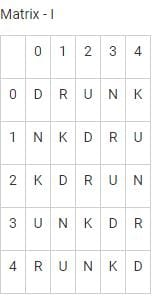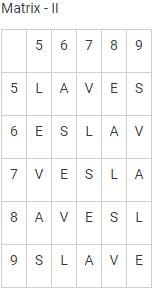Solution:

1) 33, 78, 40, 58 → DLRE

2) 21, 55, 13, 99 → DLRE

3) 44, 85, 34, 65 → DARE

4) 12, 67, 22, 87 → DLRE

Hence correct answer is 44, 85, 34, 65.

QUESTION: 32

From the given alternatives, select the word which can be formed using the letters of the given word.

CONTENTION

Solution:

Given word is CONTENTION

NATION – it can’t be formed.

TONIC – it can be formed.

OINTMENT – it can’t be formed.

STATION – it can’t be formed.

Hence, only TONIC is formed from given word.

QUESTION: 33

Identify the diagram that best represents the relationship among the classes given below:

Rat, Mammal, Eagle

Solution:
QUESTION: 34

In the following question, the symbols %, #, & and @ are used with the following meaning as illustrated below:

L % M means L is son of M.

L # M means L is daughter of M.

L & M means L is brother of M.

L @ M means L is sister of M.

If D % B # C @ A, then how is A related to D?

Solution:
QUESTION: 35

In the given diagram, Circle represents tall men, Square represents heavy men and Triangle represents generals. Which region represents generals who are heavy but not tall?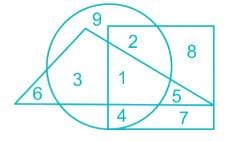Solution:

We require the part common between triangle and square, but not the circle. Therefore, the required region is denoted by 5.

QUESTION: 36

Arrange the following words as per order in the dictionary.

(1)  Acarpous
(2) Across
(3) Accede
(5) Accenture

Solution:

Solution: According to the Dictionary, the given words can be arranged as:

1. Acarpous

3. Accede

5. Accenture

2. Across

Hence, the correct order is 4, 1, 3, 5, 2

QUESTION: 37

Arrange the given words in the order in which they will be arranged in a dictionary and choose the one that comes second.

1. Triggered

2. Trenchant

3. Trembling

4. Trail

Solution:

First two letters of all the words are common i.e. ‘Tr’; hence we need to look at next letter.

As per third letter, ‘A’ comes before ‘E’, ‘E’ comes before ‘I’. Hence, Trail would come first, Trenchant and Trembling would come second and third in some order, while Triggered would come last.

For Trenchant and Trembling, we need to look at fourth letter as their first three letters are same. As per fourth letter, ‘M’ would come before ‘N’; hence Trembling would come before Trenchant.

Words in alphabetical order will be Trail, Trembling, Trenchant, Triggered.

Thus, the word that comes second is Trembling.

QUESTION: 38

If 'ARATHY' is coded as 'BSBUIZ' then 'SYSTEM' should be

Solution:
QUESTION: 39

Directions:In making decisions about important questions, it is desirable to be able to distinguish between Strong arguments and weak arguments. Strong arguments are those, which are both important and directly related to the question. Weak arguments are those, which are of minor importance and also may not be directly related to the question or may be related to a trivial aspect of the question. The question below is followed by two arguments numbered I and II. You have to decide which of the arguments a strong argument is and which a Weak argument is.

Statement: Globalization has reduced patriotism of people and their love towards their motherland.

Arguments:

I. It leads to people leaving their own nations and serve other countries.

II. It makes the people forget about their own countries and makes them strive for the better.

Solution:

Both the arguments are weak. One person working in some other country does not mean serving the other country as one needs to pay proper taxes to the homeland. Patriotism has nothing to do with dreaming for the better. Thus option 4 is the correct answer.

QUESTION: 40

If ‘+’ means ‘÷’, ‘–’ means ‘x’, ‘÷’ means ‘–’ and ‘x’ means ‘+’, then,

42 ÷ 24 + 6 × 4 - 3 = ?

Solution:

42 ÷ 24 + 6 × 4 – 3 (Given Equation)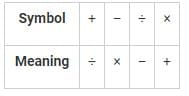⇒ Converting into Meaning symbols:

42 – 24 ÷ 6 + 4 × 3

Opening using BODMAS rule.

42 – 4 + 4 × 3

⇒ 42 – 4 + 12 = 54 – 4 = 50

QUESTION: 41

Directions: In the following question, two statements are given followed by two conclusions I and II. You have to consider the statements to be true even if they seem to be at variance from commonly which of the given conclusions, is any, follow from the given statements:

Statements:

1. Some idiots are clever.

2. All stupid’s are idiots.

Conclusions:

I. All idiots are stupid’s.

II. Some stupid’s can be clever

Solution:
QUESTION: 42

Directions: Select the missing number from the given responses.

7          13           14

4          11           13

1           9             ?

Solution:

The numbers have been decreased by 3 in the first column, 2 in the second column and 1 in the third column.

Thus the missing number is 13 – 1 = 12

QUESTION: 43

Direction: In the following question, find the missing number from the given responses.

7          9          8

4          8          6

2          3          4

30       ?          52

Solution:

The relation is as shown below

7 × 4 = 28 + 2 = 30

8 × 6 = 48 + 4 = 52

Similarly,

9 × 8 = 72 + 3 = 75

Hence, the missing number is 75.

QUESTION: 44

In the given figure, circle stands for cultural club, triangle stands for sports club and rectangle stands for music club. Show who are in music club, as well as cultural club and not in sports club: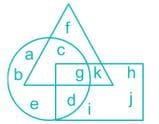Solution:

Given:-

Circle – Cultural Club

Triangle – Sports Club

Rectangle – Music Club

We need to find out those who are in Music and Cultural clubs but not in sports.

So, Circle + Rectangle – Triangle

So, ‘d’ is the one present in both circle and rectangle but not in triangle.

Hence, Option (B) is the right answer.

QUESTION: 45

How many triangles are there in the given figure?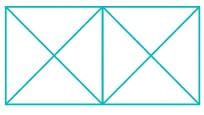Solution:
QUESTION: 46

Observe the figures and find a mirror image of the same from the alternatives given. Imagine that MN is a mirror.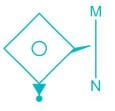Solution:
QUESTION: 47

Find the figure from alternatives in which the question figure is hidden: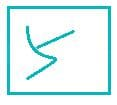Solution:
QUESTION: 48

Which answer figure will complete the pattern in the question figure?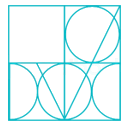Solution:
QUESTION: 49

In the question below is given a statement followed by two conclusions numbered I and II. You have to assume everything in the statement to be true, then consider the two conclusions together and decide which of them logically follows beyond a reasonable doubt from the information given in the statement.

Statement: If mileage and looks are your criteria for buying a car, Renault’s Duster is your best bet.

Conclusions:

I. Renault Duster gives good mileage.

II. It is the best looking car of all times.

Solution:

The statement suggests that Renault Duster is being suggested to someone for whom mileage and looks are important attributes. Thus, it implies that the car gives good mileage. Hence, conclusion I follows.

The second conclusion cannot be deduced because the statement does not convey any information about comparison of the car with any other contemporary car in terms of looks. Thus this conclusion does not follow.

QUESTION: 50

Which of the following cube in the answer figure cannot be made based on the unfolded cube in the question figure?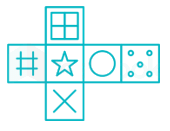Solution:Use Code STAYHOME200 and get INR 200 additional OFF Use Coupon Code